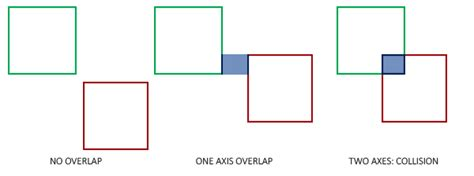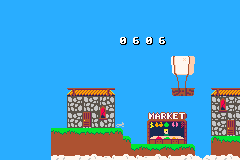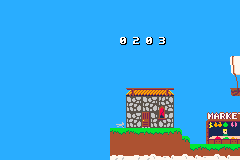[gbagamejam2021]

## GBAGameJam - Days 14-17

### Major Refactor - Classes

So. How do classes in C++ work? Yeah, that took some googling on its own. Actually just trying to figure out the weirdness of their constructors was time consuming.

I ended up making a hitbox class that I could use for my character. This is what it looks like...

``class Hitbox{    private:        int _x;        int _y;        int _width;        int _height;    public:        Hitbox(int x, int y, int width, int height)            : _x(x), _y(y), _width(width), _height(height)        {}        int x()        {            return _x;        }        int y()        {            return _y;        }        int width()        {            return _width;        }        int height()        {            return _height;        }};``

This is clearly not something that you should be learning from me though. So I will leave a few links here..

https://www.cplusplus.com/doc/tutorial/classes/

Now comes what I have been dreading, a big refactor to create a player class.

I am actually working in a few other changes at the same, just to make it even worse for me.

• Add AABB collision based on hitboxes
• Move towards a velocity based model for movement
• Add state management that I can tie animations to further down the line.

So lets try to go throught this together and hope it makes sense.

### AABB Collisions

AABB or Axis-Aligned Bounding Box is a simple way of detecting whether two rectangular objects are overlapping (assuming that neither is rotated - thus aligned). This works well for a lot of simple gameplay mechanics. And also why I introduced hitboxes on our player.

Could I have just used the sprite size as the hitbox? Yes. But you can get much better precision by making your own, especially good if your character doesn't take up the whole 8x8 sprite size.To do this I find the X component for the left and right sides and the Y component for both the top and bottom.

``int l = pos.x().integer() - hitbox.width() / 2 + hitbox.x();int r = pos.x().integer() + hitbox.width() / 2 + hitbox.x();int u = pos.y().integer() - hitbox.height() / 2 + hitbox.y();int d = pos.y().integer() + hitbox.height() / 2 + hitbox.y();``

NOTE: The width and height are divided by two because the sprites pos is in it's centre.

Then I use those to determine what the player is colliding with at a point in time.

So `get_map_cell(l, u, map)` will return the map cell that the top left corner is interacting with.

We use this in a way where if any of the corners overlap with any of the 'floor' tiles, then it is considered a collision. If it collides the player is placed at the top of the tile and dy (vertical velocity) is set to 0.

``if(get_map_cell(l, u, map) < 13) ||  get_map_cell(l, d, map) < 13 ||  get_map_cell(r, u, map) < 13 ||  get_map_cell(r, d, map) < 13){    return true;} else {    return false;}``

And I made a fall() function that is applied every update...

``void fall(bn::affine_bg_ptr map){    if(check_fall_collisions(_pos, _hitbox, map)){        if(_dy > 0)        {            _dy = 0; // set vertical velocity to 0            if(!_grounded){ // if we aren't already on the ground then lets move us to the top and set our flag                _pos.set_y(_pos.y() - modulo(_pos.y() + 4,8));                _grounded = true;            }        } else {            // character is jumping, lets not mess with this        }    } else { // otherwise we are falling and not grounded        _dy+= gravity;         _grounded = false;    }}``

Boom collisions done.If you noticed we also added a `_grounded` flag to our player, this will later be tied to player animation states.

### Acceleration and Velocity

You might also notice that above we are now using dy to represent the delta of y (y velocity). This way we can do cool things like have the character accelerate from 0 to their max speed, rather than instantly be moving.

Our `move_right()` function now flips the sprite and adds to the velocity rather than the position..

``void move_right(){    _sprite.set_horizontal_flip(false);    _dx+= acc;}``

Then we go to actually move the character we do a few different things...

``// apply friction_dx = _dx * friction;_dy = _dy * friction;//apply limitsif( _dx > max_dx){    _dx = max_dx;}if( _dy > max_dy){    _dy = max_dy;}// update position_pos.set_x(_pos.x() + _dx);_pos.set_y(_pos.y() + _dy);``

As you can see we have a constant friction variable now that is applied to slow the character down if they aren't moving. We also clamp to a max speed in each direction. And lastly we apply our new dy and dx to the players current position.

This is an exaggerated example of this effect...The code is kind of all over the place right now, but feel free to take a look at today's tag day-17.

.gba

Previous Post - Collisions, Music and More
Next Post - Animation States and Dash Ability

Want to get notified next time I post?